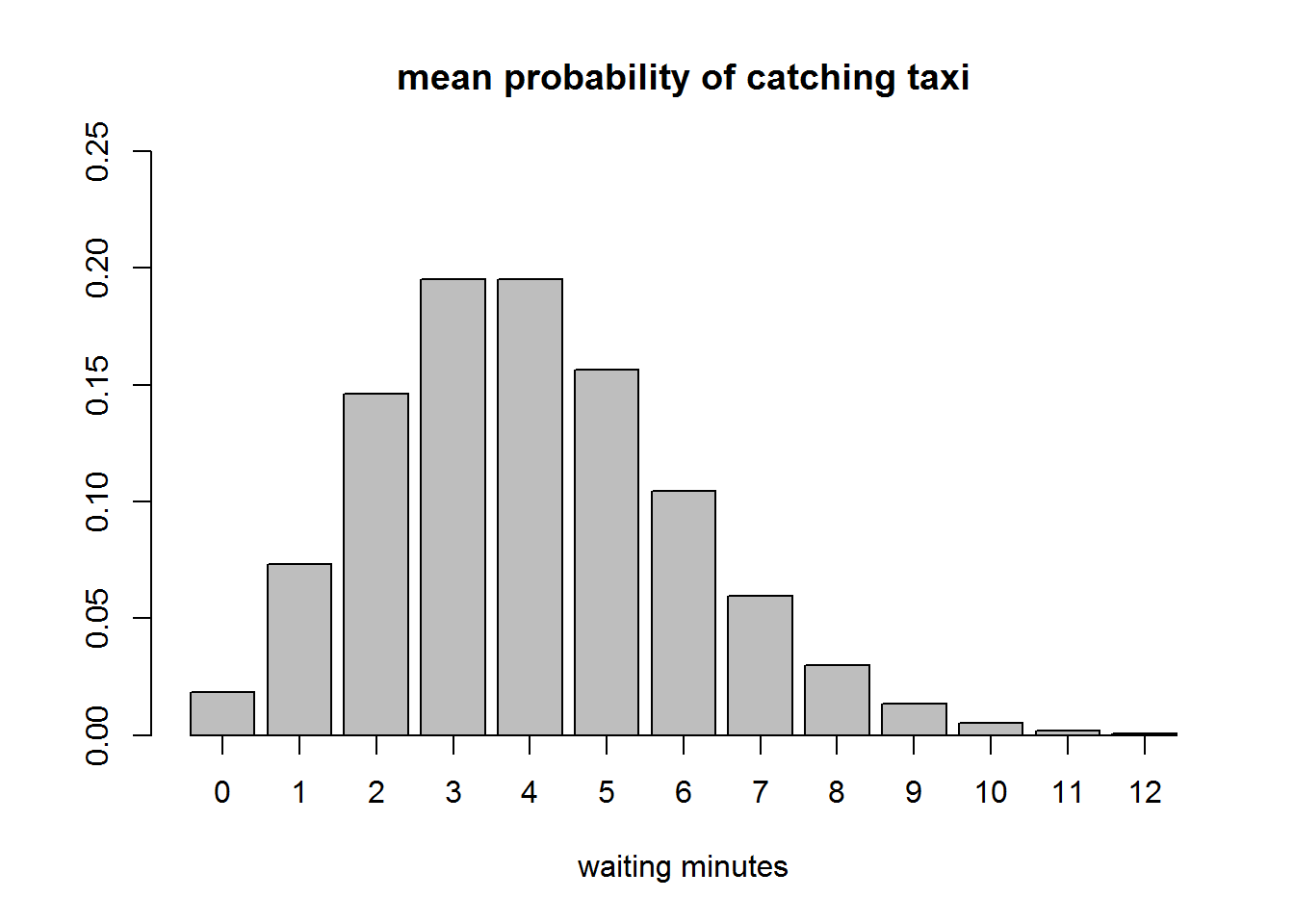Refs:

Information theory provides a constructive criterion for setting up probability distributions on the basis of partial knowledge, and leads to a type of statistical inference which is called the maximum entropy estimate. It is least biased estimate possible on the given information; i.e., it is maximally noncommittal with regard to missing information. [ET. Jaynes, 1957]

The entropy of a distribution $$p(n)$$ is

$H(p) = - \sum_i p(i) \log p(i)$

if discrete, or if continuous

$H(p) = - \int_x p(x) \log p(x) dx$

The maximum entropy principle (MaxEnt) states that the most appropriate distribution to model a given set of data is the one with highest entropy among all those that satisfy the constrains of our prior knowledge.

Usually, these constrains are given as equations regarding moments of the desired distribution. Given functions $$f_1, \ldots, f_K$$ and numbers $$a_1, \ldots, a_K$$, the constrains are of form

$E_p[f_k(X)] = \sum_{n=1}^N f_k(n) p(n) = a_k, ~~~k=1, \ldots, K,~~X \sim p$

for the discrete case.

If there is a solution which is a distribution (sum/integrate to 1) and is the maximal entropy solution, then this (unique) distribution has form

$p(n) = \frac{1}{Z} \exp \left( - \sum_k \lambda_k f_k(n) \right)$

where $$Z$$ is the normalization value

$Z = \sum_n \exp \left( - \sum_k \lambda_k f_k(n) \right)$

and $$\lambda_k$$ are appropriate real values usually found by Lagrange multipliers.

For instance, if the only constraint is $$f_1(n) = \log(n)$$, the expression will be

$p(n) \propto \exp \left( -\lambda_1 \log(n) \right) = n^{-\lambda_1}$

which describes a power-law distribution (we would need to find $$\lambda_1$$’s value).

Some egs:

• The uniform is the maxent distribution if we only know that valid values are within an interval $$[a,b]$$; its entropy is $$\log(b-a)$$

• The normal distribution is the maxext with support $$(-\infty,+\infty)$$ when the constraints are $$f_1(n)=n$$ (ie, the mean) and $$f_2(n)=n^2$$ (proportional to the variance, since $$Var(X) = E[X^2] - (E[X])^2$$); its entropy is $$\log(\sigma \sqrt{2\pi e})$$.

• The exponential distribution, with parameter $$\lambda$$, is the maxext with support $$[0,+\infty)$$ when the constraints are $$f_1(n)=n$$; its entropy is $$1-\log\lambda$$.
• The log-normal distribution is the maxext with support $$[0,+\infty)$$ when the constraints are $$f_1(n)=\log(n)$$ and $$f_2(n)=\log(n)^2$$; its entropy is $$\mu + 0.5 \log(2\pi e \sigma^2)$$

• The Pareto distribution, with parameters $$x_m>0, \alpha>0$$ (a power-law distribution) with support $$[x_m,+\infty)$$ when the constrains are $$f_1(n)=\log(n)$$; its entropy is $$\log(x_m/\alpha) + 1/\alpha + 1$$.

More distributions and details here and here.

We can also approximate the entropy of a generic continuous distribution using integrate:

# for continuous functions
entropy_approx <- function(f, lower, upper, ...) {
- integrate(function(x, ...) f(x, ...) * log(f(x, ...)), # - \int f(x) log f(x) dx
lower = lower,
upper = upper,
...)$value } ### approximating the Normal entropy p_mu = 0 p_sd = 1 H_normal <- entropy_approx(dnorm, lower = p_mu - 10*p_sd, upper = p_mu + 10*p_sd, mean = p_mu, sd = p_sd) # comparing with correct value log(p_sd* sqrt(2*pi*exp(1))) ##  1.418939 H_normal ##  1.418939 ### approximating the Exponential entropy lambda <- 2 H_exp <- entropy_approx(dexp, lower = 0, upper = 1e2, rate = lambda) # comparing with correct value 1- log(lambda) ##  0.3068528 H_exp ##  0.3068528 ## MaxEnt Approximation by Optimization We can apply optimization with the necessary constraints to get approximate discrete distribution to the theoritical MaxEnt solutions. The next optimization is based on this example. Extra software/packages needed: • Matlab • CVX Matlab package • CVXfromR R package: to install run at R’s cmd: install.packages("C:/{your path}/CVXfromR_1.6.zip", repos = NULL, type="binary") The optimization program will have the following structure: Maximize the vector pmaxent coding the probability mass of the discrete distribution, considering the constraints sum(pmaxent)==1 that keeps the solution has a distribution, and A*pmaxent == b where matrix A has, on each row, a transformation $$f_i$$ and the respective index of b has the constraint value. Example 1: let’s constrained the solution to $$E[X] = \frac{1}{\lambda}$$ and check that we will get an approximation to the exponential distribution $$\exp(\lambda)$$: library(CVXfromR) n <- 100 a <- seq(0,10,len=n) # theoretical support [0,+oo) but we assume a light tail lambda <- .5 A <- matrix(a, ncol=n) b <- 1/lambda # f_1(n) = n, ie, E[f_1(n)] = E[n] = 1/lambda # ref: web.cvxr.com/cvx/examples/cvxbook/Ch07_statistical_estim/html/maxent.html # entr(x)=-x*log(x), elementwise entropy function [cvxr.com/cvx/doc/funcref.html] cvxcode <- " variables pmaxent(n) maximize( sum(entr(pmaxent)) ) sum(pmaxent) == 1; A * pmaxent == b; " # it takes sometime to run a matlab session opt.vals <- CallCVX(cvxcode, const.vars=list(n=n, A=A, b=b), opt.var.names="pmaxent", setup.dir="C:\\Users\\jpn.INFORMATICA\\Software\\_Langs\\cvx") plot(a,opt.vals$pmaxent, pch=20, ylab="")
diff <- dexp(0,rate=lambda) / opt.vals$pmaxent # scale back to maxent approx curve(dexp(x,rate=lambda)/diff, col="red", add=T)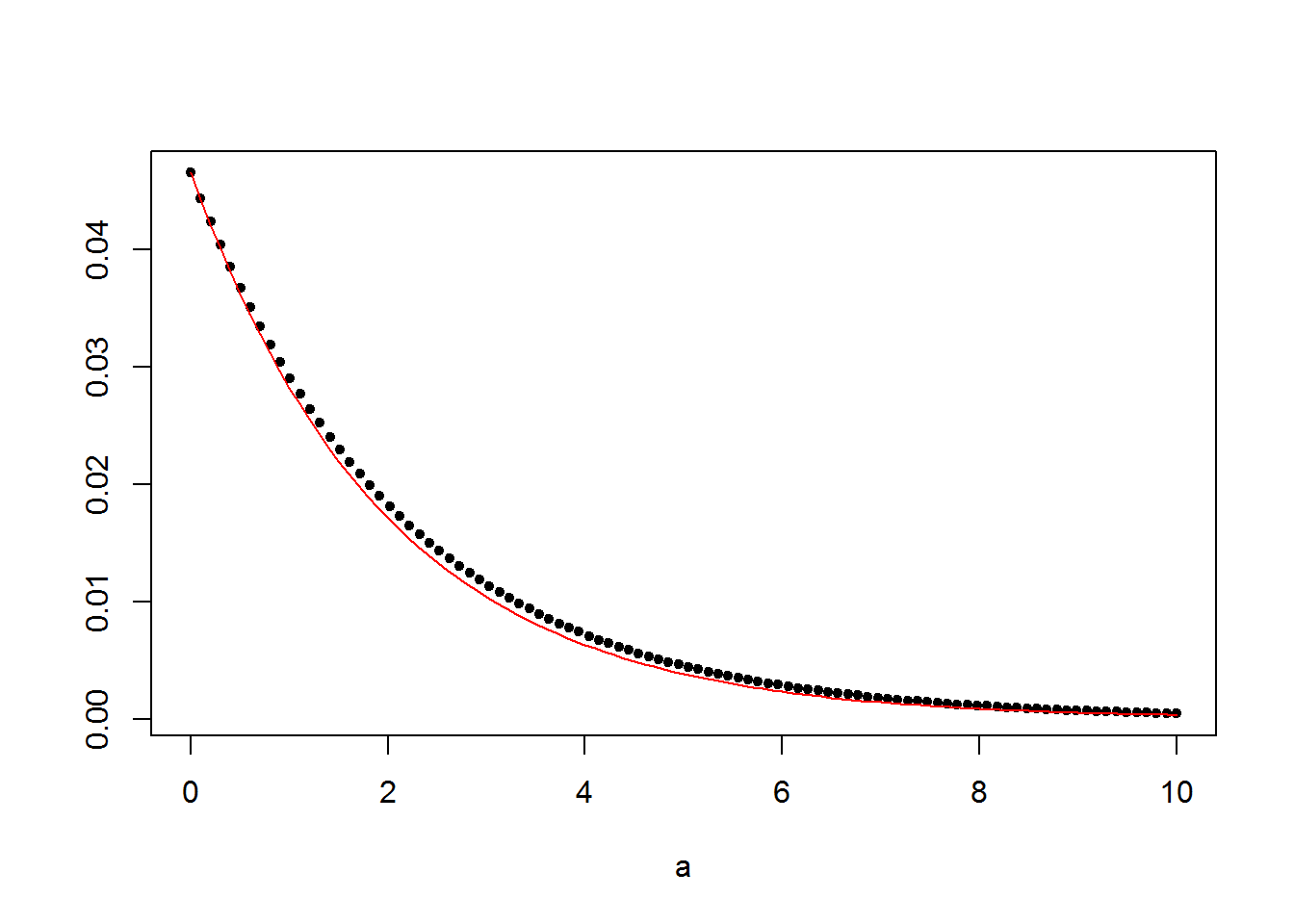Notice that the vector opt.vals$pmaxent describes a discrete distribution that approximates the exponential continuous distribution. The scaling is just to translate the exponential’s pdf value (ie, a density) into the magnitude of the discrete pmf values.

Example 2: let’s try now by constraining the $$E[X] = 1$$ and $$E[X^2] = 0$$ and check that we will get an approximation to the standard normal distribution $$\mathcal{N}(0,1)$$:

n  <- 100
a  <- seq(-3,3,len=n) # support is (-oo,+oo) but here's 99% distribution's mass
a2 <- a^2

A <- matrix(c(a,a2), ncol=n, byrow=TRUE)
b <- c(0,           # f_1(n) = n,   ie, E[f_1(n)] = E[n]   = 0
1)           # f_2(n) = n^2, ie, E[f_2(n)] = E[n^2] = 1

opt.vals <- CallCVX(cvxcode, const.vars=list(n=n, A=A, b=b),
opt.var.names="pmaxent",
setup.dir="C:\\Users\\jpn.INFORMATICA\\Software\\_Langs\\cvx")

plot(a,opt.vals$pmaxent, pch=20, ylab="") diff <- max(dnorm(a))/max(opt.vals$pmaxent) # scale back to maxent approx
curve(dnorm(x)/diff, col="red", add=T)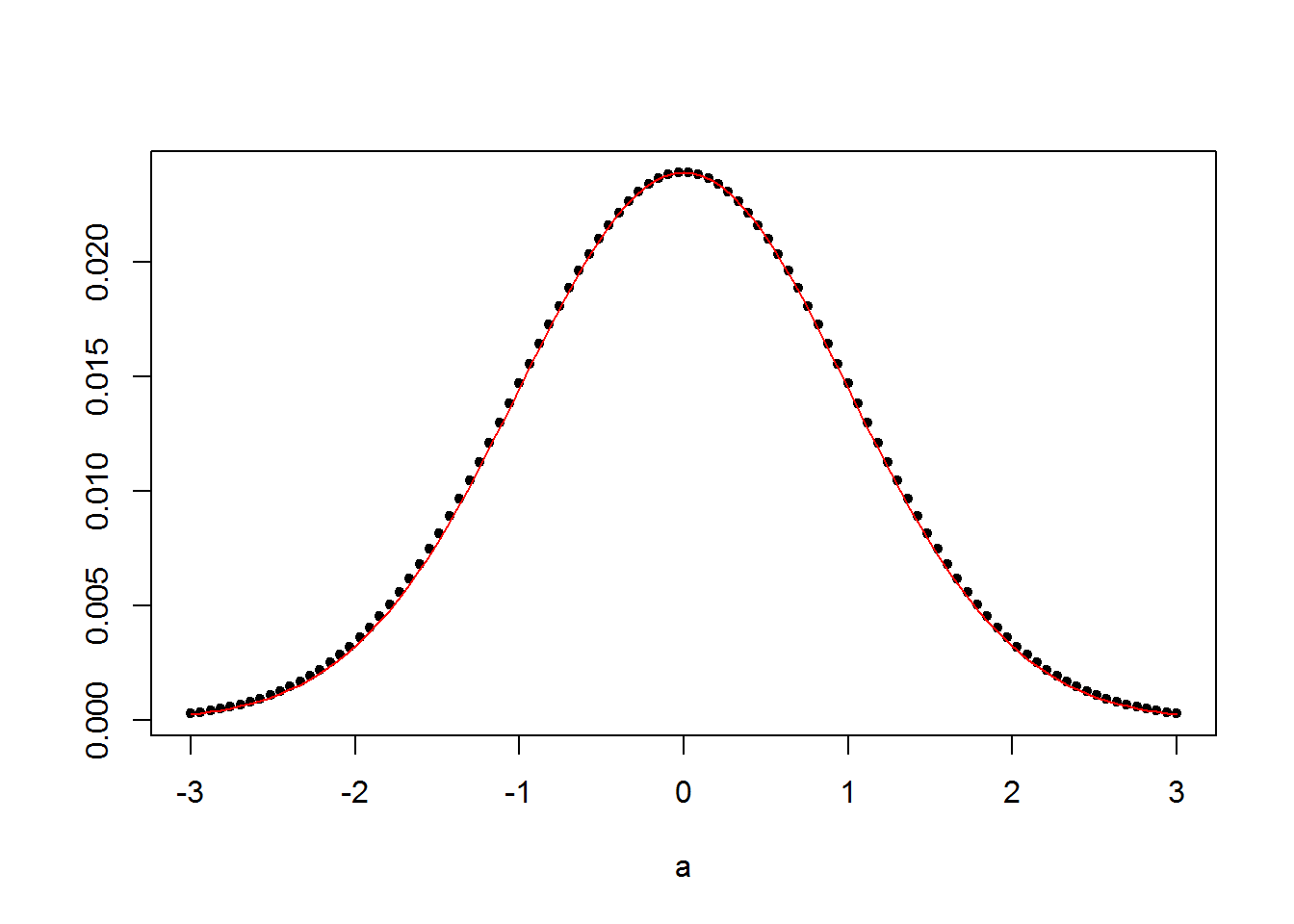A log-normal eg:

n  <- 100
a  <- seq(1e-3,10,len=n) # support is [0,+oo)
log_mu <- 1
log_sd <- 0.5

log_a  <- log(a)
log_a2 <- (log(a)-log_mu)^2

A <- matrix(c(log_a,log_a2), ncol=n, byrow=TRUE)
b <- c(log_mu,       # E[log(n)]        = mu
log_sd^2)     # E[(log(n)-mu)^2] = sd^2

opt.vals <- CallCVX(cvxcode, const.vars=list(n=n, A=A, b=b),
opt.var.names="pmaxent",
setup.dir="C:\\Users\\jpn.INFORMATICA\\Software\\_Langs\\cvx")

plot(a,opt.vals$pmaxent, pch=20, ylab="") # scale back; exp(log_mu-log_sd^2) is the mode of the log-normal diff <- dlnorm(exp(log_mu-log_sd^2),log_mu,log_sd)/max(opt.vals$pmaxent)
curve(dlnorm(x,log_mu,log_sd)/diff, col="red", add=T)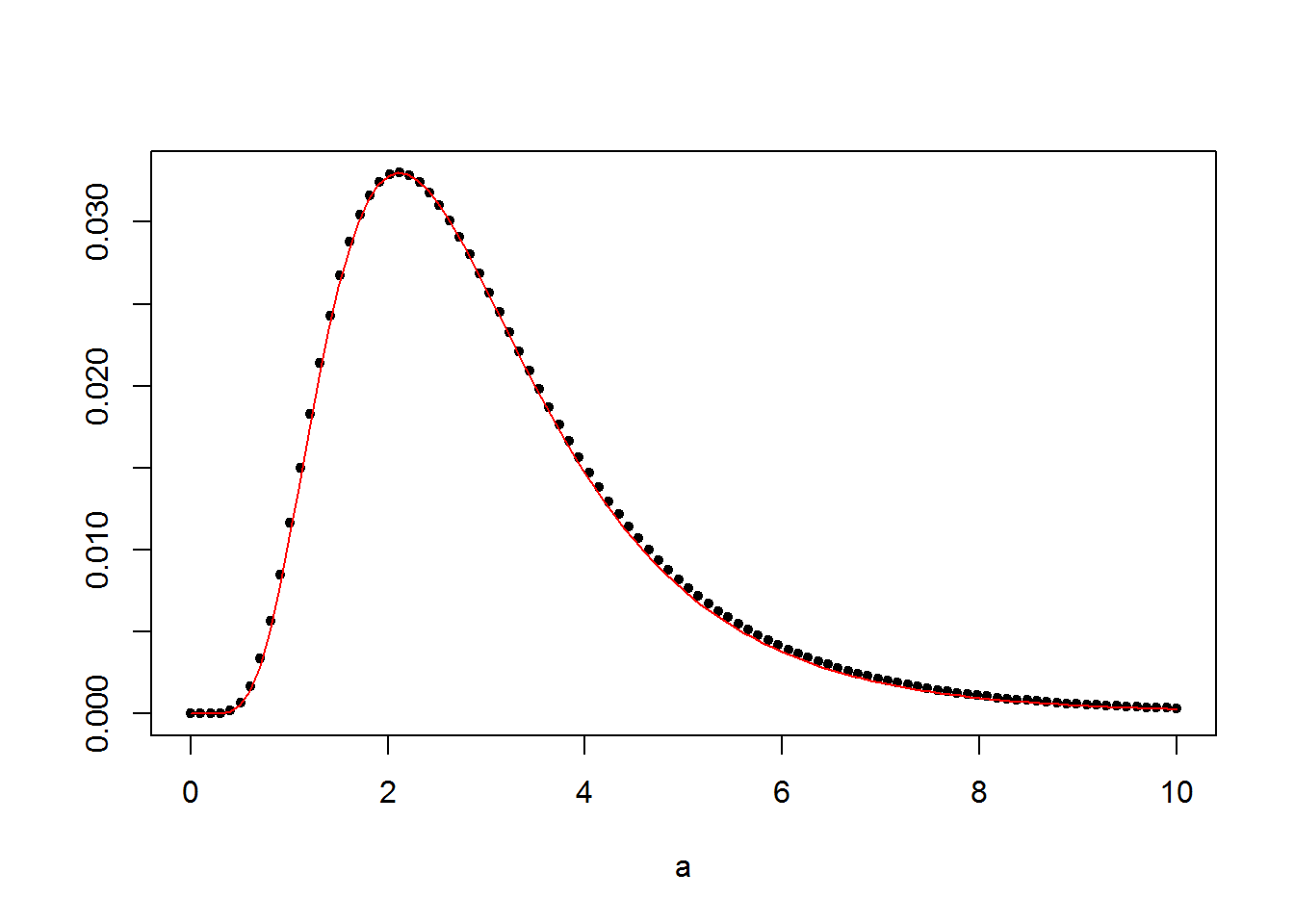And if we remove the previous 2nd constraint, we get a Pareto distribution (an eg of power-law):

n  <- 200
pareto_alpha <- 1.2
pareto_xm    <- 1
# support is [xm,+oo), need to extend the upper limit due to its heavy tail
# otherwise, the optimization will put excessive probability mass at the beginning
a  <- seq(pareto_xm,40,len=n)

log_a  <- log(a)

A <- matrix(c(log_a), ncol=n, byrow=TRUE)
b <- c(1/pareto_alpha + log(pareto_xm))

opt.vals <- CallCVX(cvxcode, const.vars=list(n=n, A=A, b=b),
opt.var.names="pmaxent",
setup.dir="C:\\Users\\jpn.INFORMATICA\\Software\\_Langs\\cvx")

plot(a,opt.vals$pmaxent, xlim=c(pareto_xm,pareto_xm+10), pch=20, ylab="") # scale back; xm is the mode of the Pareto diff <- pareto_xm/max(opt.vals$pmaxent)
library(VGAM)
## Loading required package: stats4
## Loading required package: splines
curve(dpareto(x, pareto_xm, pareto_alpha)/diff, col="red", add=T)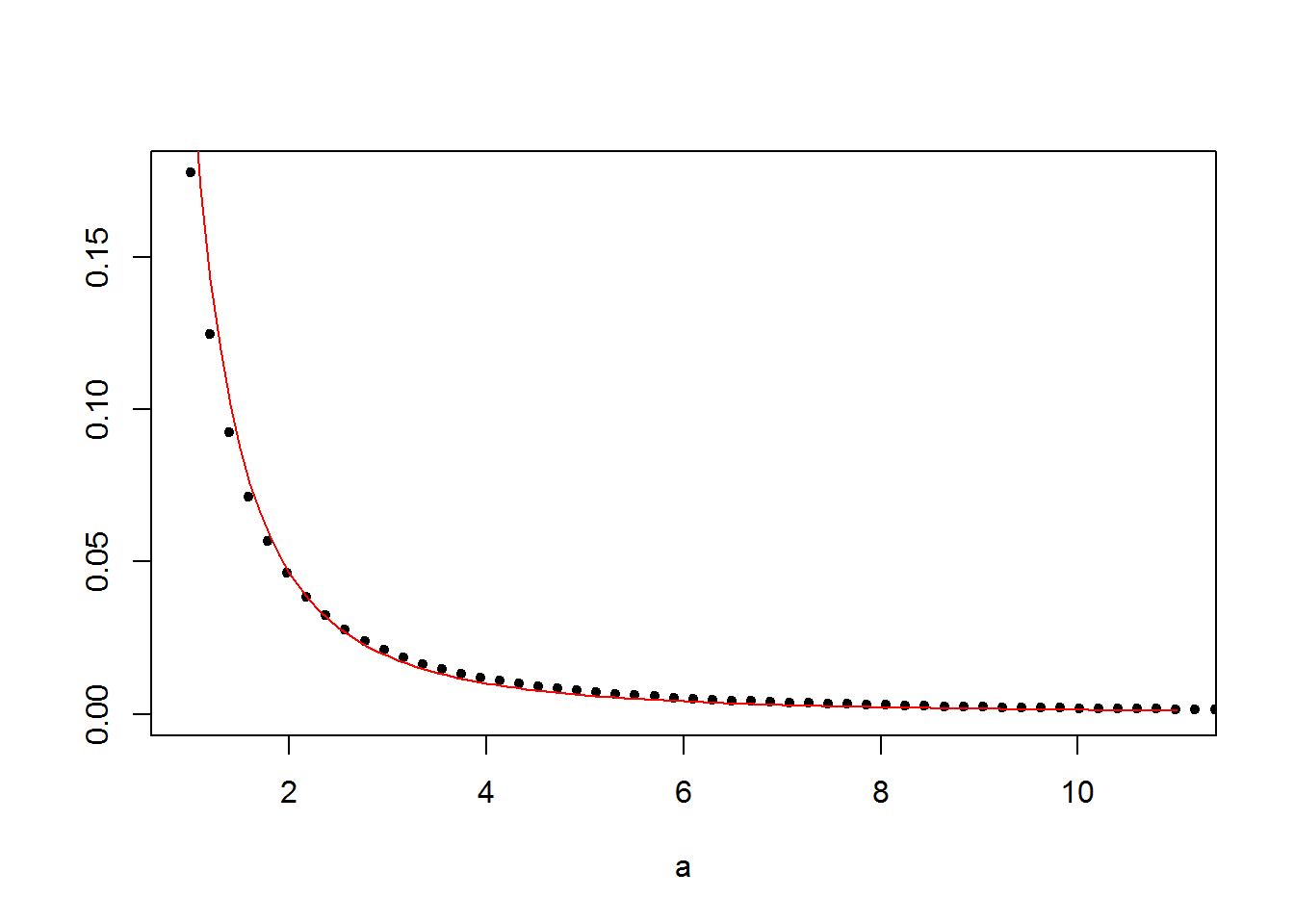This use of CVX allow us to build maxent solutions for more complex constraints. This next is the original eg converted to R:

# We consider a probability distribution on 100 equidistant points in the
# interval [-1,1]. We impose the following prior assumptions:
#
#    -0.1 <= E(X) <= +0.1
#    E[X^2] == 0.5  <==> +0.5 <= E(X^2) == +0.5
#    -0.3 <= E(3*X^3-2*X) <= -0.2
#    +0.3 <= Pr(X<0) <= 0.4

n <- 100
a  <- seq(-1,1,len=n)

a2 <- a^2
a3 <- 3*a^3-2*a;
ap <- 0+(a<0)

A <- matrix(c( a, -a, a2,-a2, a3,-a3, ap,-ap), ncol=n, byrow=TRUE)
b <-        c(.1, .1, .5,-.5,-.2, .3, .4,-.3)

cvxcode <- "
variables pmaxent(n)
maximize( sum(entr(pmaxent)) )
sum(pmaxent) == 1;
A * pmaxent <= b;
"

opt.vals <- CallCVX(cvxcode, const.vars=list(n=n, A=A, b=b),
opt.var.names="pmaxent",
setup.dir="C:\\Users\\jpn.INFORMATICA\\Software\\_Langs\\cvx")

plot(a,opt.vals$pmaxent, type="l", lwd=2, ylab="", main="maxent distribution")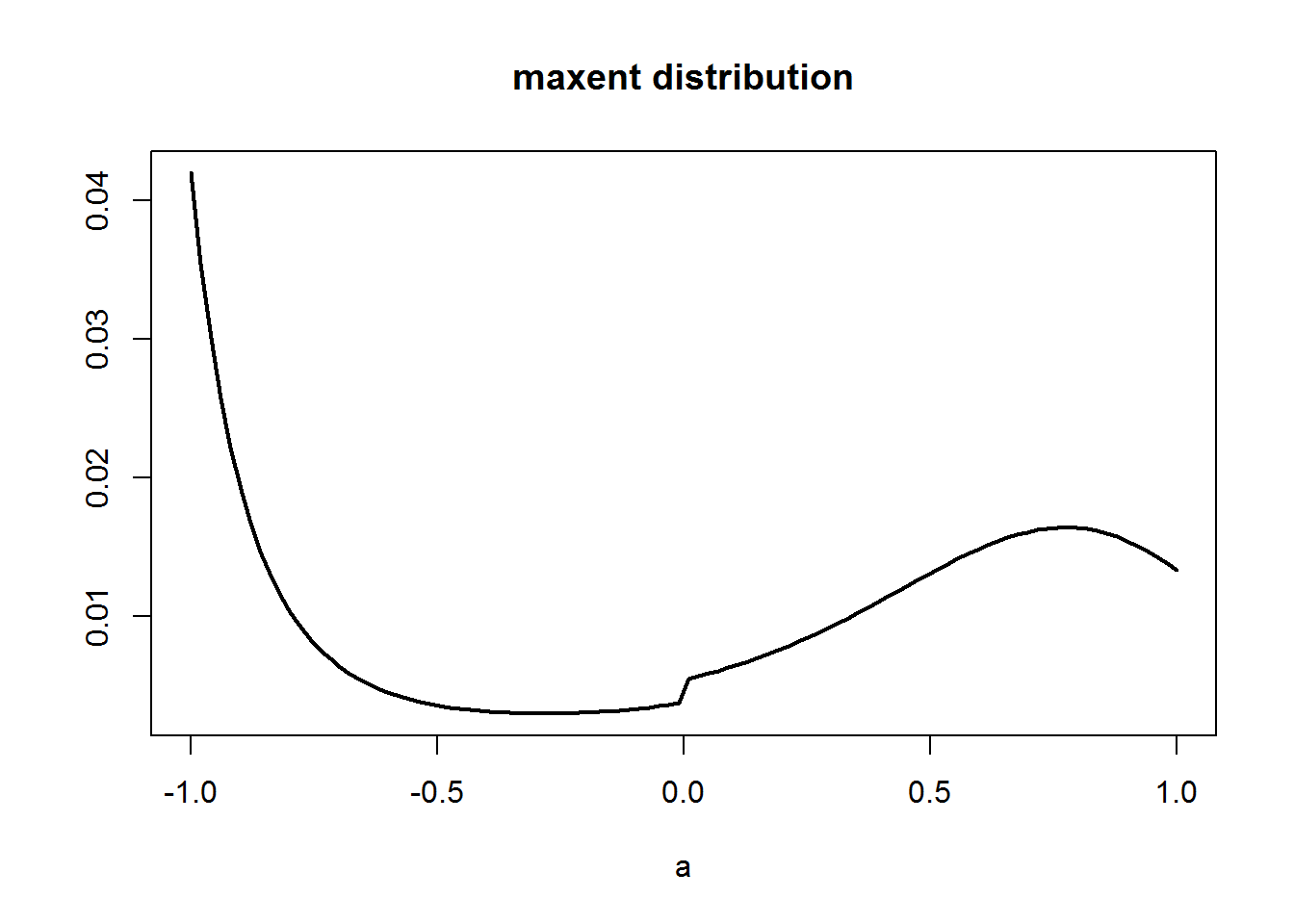## Using MaxEnt to build a prior for bayesian inference taxi <- c(6,3,4,6,2,3,2,6,4,4) # waiting times in minutes mean(taxi) ##  4 In this case we wish to find between all distributions that could have generated the previous vectors – there are infinite – the one with highest entropy. To narrow the search, we include a constraint stating that distribution should have expected value of $$4$$, the mean of our sample. Using the method of Lagrange multipliers it’s possible to find that the distribution solution is given by: $p(x) = (1-\exp(-\lambda)) \exp(-\lambda x)$ where $$\lambda \approx 0.22$$ (cf. details here and here) # maxent solution pmf_maxent <- function(x,lambda=0.22) (1-exp(-lambda))*exp(-lambda*x) sum(pmf_maxent(0:100)) # check if it's a distribution ##  1 mp <- barplot(pmf_maxent(0:15), ylim=c(0,.25), xlab="waiting minutes") axis(1,at=mp,labels=paste(0:15))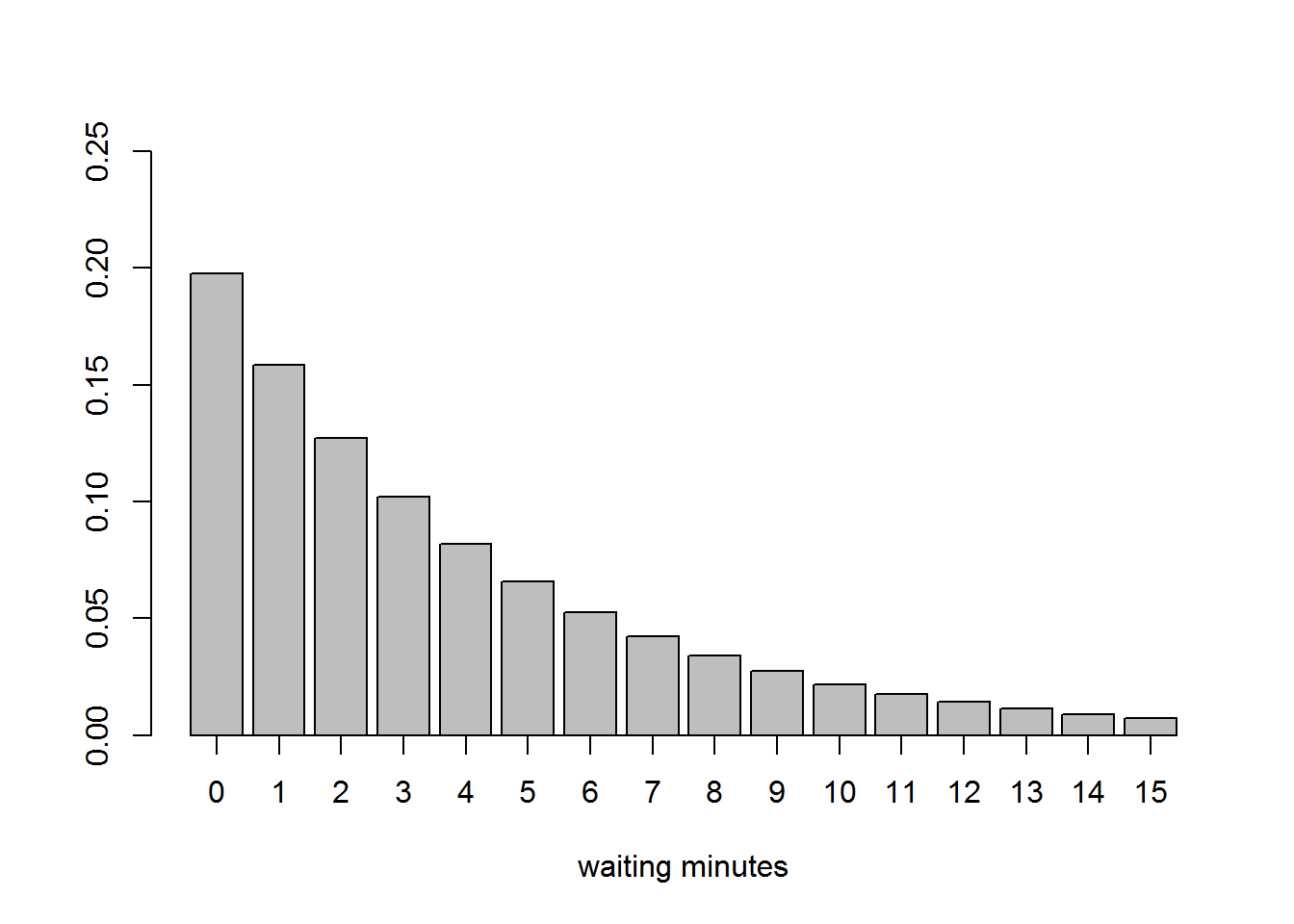This could be treated has a prior for making a bayesian analysis. Notice that we would use some information about the data to define this prior, namely its expected value (not much information but still). To simplify, we’ll use a fitted exponential to approximate the maxent proposal: sample_taxi <- rep(0:40, round(pmf_maxent(0:40)*1e3,0)) # translate histogram to values library('fitdistrplus') fit <- fitdist(sample_taxi, "exp") # fit exponential to sample fit$estimate
##      rate
## 0.2480119

So, the value of $$\lambda$$ is 0.2480119:

mp <- barplot(pmf_maxent(0:15), ylim=c(0,.25), xlab="waiting minutes")
axis(1,at=mp,labels=paste(0:15))
points(as.vector(mp), dexp(as.vector(mp),fit$estimate), lwd=2, col="red", type="l")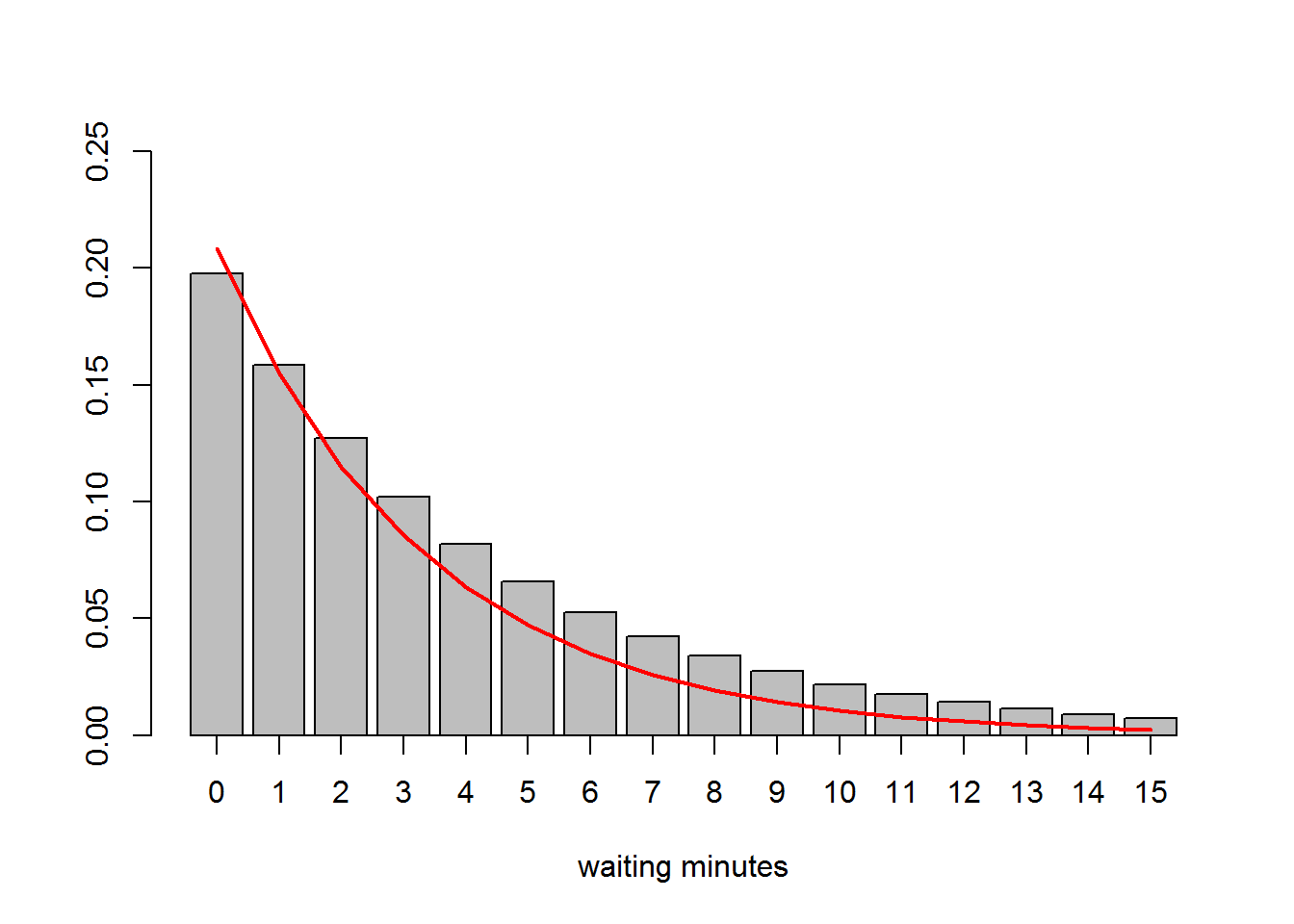Here we used the poisson for the likelihood. The model in BUGS and its execution: modelString = " model { theta ~ dexp(lambda) for(i in 1:N) { taxi[i] ~ dpois(theta) } } " data.list = list( taxi = taxi, N = length(taxi), lambda = fit$estimate
)

run.model(modelString, samples=c("theta"), data=data.list, chainLength=1e4)
## model is syntactically correct
## data loaded
## model compiled
## initial values generated, model initialized
## 1000 updates took 0 s
## monitor set for variable 'theta'
## 10000 updates took 0 s
report <- samplesStats("theta")
report
##        mean     sd MC_error val2.5pc median val97.5pc start sample
## theta 4.003 0.6232 0.006128    2.849  3.974     5.293  1001  10000
thetas <- samplesSample( "theta" )
hist(thetas, breaks=30, main=expression(paste("sampling of ", theta)), yaxt='n', ylab="")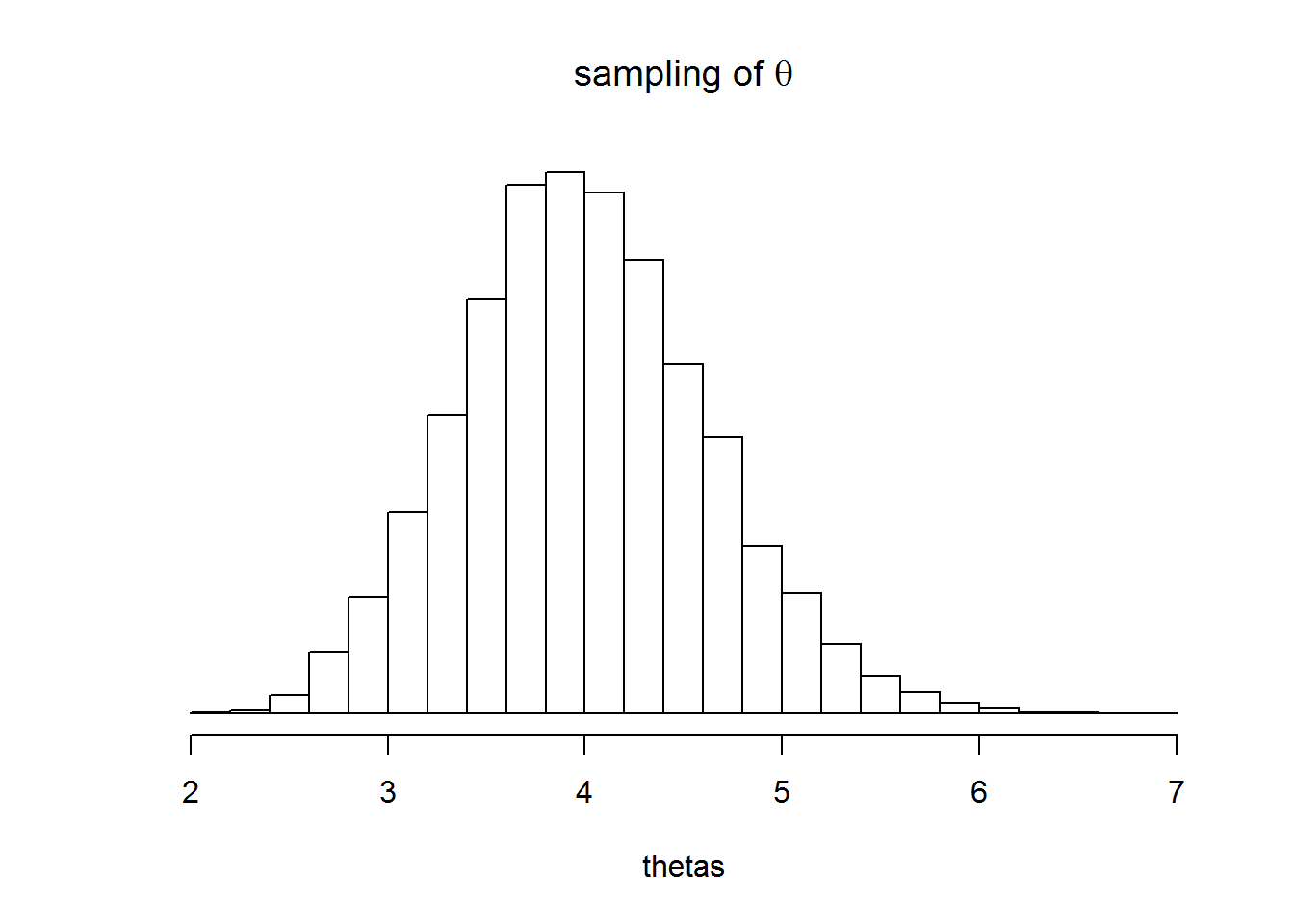mp <- barplot(dpois(0:12,report\$mean), xlab="waiting minutes", ylim=c(0,.25))
axis(1,at=mp,labels=paste(0:12))
title("mean probability of catching taxi")